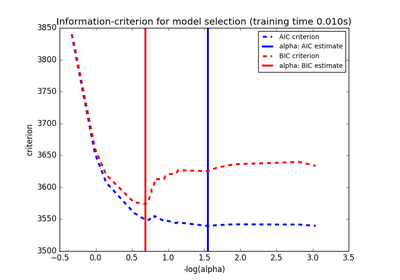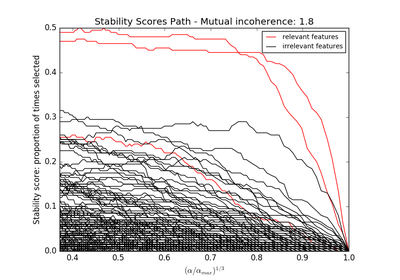# 3.2.4.1.4. `sklearn.linear_model`.LassoLarsCV¶

class `sklearn.linear_model.``LassoLarsCV`(fit_intercept=True, verbose=False, max_iter=500, normalize=True, precompute='auto', cv=None, max_n_alphas=1000, n_jobs=1, eps=2.2204460492503131e-16, copy_X=True, positive=False)[source]

Cross-validated Lasso, using the LARS algorithm

The optimization objective for Lasso is:

```(1 / (2 * n_samples)) * ||y - Xw||^2_2 + alpha * ||w||_1
```

Read more in the User Guide.

Parameters: fit_intercept : boolean whether to calculate the intercept for this model. If set to false, no intercept will be used in calculations (e.g. data is expected to be already centered). positive : boolean (default=False) Restrict coefficients to be >= 0. Be aware that you might want to remove fit_intercept which is set True by default. Under the positive restriction the model coefficients do not converge to the ordinary-least-squares solution for small values of alpha. Only coefficients up to the smallest alpha value (```alphas_[alphas_ > 0.].min()``` when fit_path=True) reached by the stepwise Lars-Lasso algorithm are typically in congruence with the solution of the coordinate descent Lasso estimator. As a consequence using LassoLarsCV only makes sense for problems where a sparse solution is expected and/or reached. verbose : boolean or integer, optional Sets the verbosity amount normalize : boolean, optional, default False If True, the regressors X will be normalized before regression. This parameter is ignored when fit_intercept is set to False. When the regressors are normalized, note that this makes the hyperparameters learnt more robust and almost independent of the number of samples. The same property is not valid for standardized data. However, if you wish to standardize, please use preprocessing.StandardScaler before calling fit on an estimator with normalize=False. precompute : True | False | ‘auto’ | array-like Whether to use a precomputed Gram matrix to speed up calculations. If set to `'auto'` let us decide. The Gram matrix can also be passed as argument. max_iter : integer, optional Maximum number of iterations to perform. cv : int, cross-validation generator or an iterable, optional Determines the cross-validation splitting strategy. Possible inputs for cv are: None, to use the default 3-fold cross-validation, integer, to specify the number of folds. An object to be used as a cross-validation generator. An iterable yielding train/test splits. For integer/None inputs, `KFold` is used. Refer User Guide for the various cross-validation strategies that can be used here. max_n_alphas : integer, optional The maximum number of points on the path used to compute the residuals in the cross-validation n_jobs : integer, optional Number of CPUs to use during the cross validation. If `-1`, use all the CPUs eps : float, optional The machine-precision regularization in the computation of the Cholesky diagonal factors. Increase this for very ill-conditioned systems. copy_X : boolean, optional, default True If True, X will be copied; else, it may be overwritten. coef_ : array, shape (n_features,) parameter vector (w in the formulation formula) intercept_ : float independent term in decision function. coef_path_ : array, shape (n_features, n_alphas) the varying values of the coefficients along the path alpha_ : float the estimated regularization parameter alpha alphas_ : array, shape (n_alphas,) the different values of alpha along the path cv_alphas_ : array, shape (n_cv_alphas,) all the values of alpha along the path for the different folds cv_mse_path_ : array, shape (n_folds, n_cv_alphas) the mean square error on left-out for each fold along the path (alpha values given by `cv_alphas`) n_iter_ : array-like or int the number of iterations run by Lars with the optimal alpha.

Notes

The object solves the same problem as the LassoCV object. However, unlike the LassoCV, it find the relevant alphas values by itself. In general, because of this property, it will be more stable. However, it is more fragile to heavily multicollinear datasets.

It is more efficient than the LassoCV if only a small number of features are selected compared to the total number, for instance if there are very few samples compared to the number of features.

Methods

 `decision_function`(\*args, \*\*kwargs) DEPRECATED: and will be removed in 0.19. `fit`(X, y) Fit the model using X, y as training data. `get_params`([deep]) Get parameters for this estimator. `predict`(X) Predict using the linear model `score`(X, y[, sample_weight]) Returns the coefficient of determination R^2 of the prediction. `set_params`(\*\*params) Set the parameters of this estimator.
`__init__`(fit_intercept=True, verbose=False, max_iter=500, normalize=True, precompute='auto', cv=None, max_n_alphas=1000, n_jobs=1, eps=2.2204460492503131e-16, copy_X=True, positive=False)[source]
`decision_function`(*args, **kwargs)[source]

DEPRECATED: and will be removed in 0.19.

Decision function of the linear model.

Parameters: X : {array-like, sparse matrix}, shape = (n_samples, n_features) Samples. C : array, shape = (n_samples,) Returns predicted values.
`fit`(X, y)[source]

Fit the model using X, y as training data.

Parameters: X : array-like, shape (n_samples, n_features) Training data. y : array-like, shape (n_samples,) Target values. self : object returns an instance of self.
`get_params`(deep=True)[source]

Get parameters for this estimator.

Parameters: deep : boolean, optional If True, will return the parameters for this estimator and contained subobjects that are estimators. params : mapping of string to any Parameter names mapped to their values.
`predict`(X)[source]

Predict using the linear model

Parameters: X : {array-like, sparse matrix}, shape = (n_samples, n_features) Samples. C : array, shape = (n_samples,) Returns predicted values.
`score`(X, y, sample_weight=None)[source]

Returns the coefficient of determination R^2 of the prediction.

The coefficient R^2 is defined as (1 - u/v), where u is the regression sum of squares ((y_true - y_pred) ** 2).sum() and v is the residual sum of squares ((y_true - y_true.mean()) ** 2).sum(). Best possible score is 1.0 and it can be negative (because the model can be arbitrarily worse). A constant model that always predicts the expected value of y, disregarding the input features, would get a R^2 score of 0.0.

Parameters: X : array-like, shape = (n_samples, n_features) Test samples. y : array-like, shape = (n_samples) or (n_samples, n_outputs) True values for X. sample_weight : array-like, shape = [n_samples], optional Sample weights. score : float R^2 of self.predict(X) wrt. y.
`set_params`(**params)[source]

Set the parameters of this estimator.

The method works on simple estimators as well as on nested objects (such as pipelines). The latter have parameters of the form `<component>__<parameter>` so that it’s possible to update each component of a nested object.

Returns: self :

## 3.2.4.1.4.1. Examples using `sklearn.linear_model.LassoLarsCV`¶Lasso model selection: Cross-Validation / AIC / BICSparse recovery: feature selection for sparse linear models